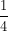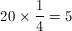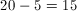## Example Questions

### Example Question #1 : Operations With Fractions

If xy = 1 and 0 < x < 1, then which of the following must be true?

< 1

x

y = 1

y > 1

y = x

y > 1

Explanation:

If x is between 0 and 1, it must be a proper fraction (e.g., ½ or ¼). Solving the first equation for y, y = 1/x. When you divide 1 by a proper fraction between 0 and 1, the result is the reciprocal of that fraction, which will always be greater than 1.

To test this out, pick any fraction. Say x = ½. This makes y = 2.

### Example Question #2 : How To Multiply Fractions

Before going to school, Joey ran 1/3 of his daily total miles. In gym class, Joey did 2/3 of the remainder. What part of his daily total miles was left for after school?

1/3

2/3

4/9

7/9

2/9

2/9

Explanation:

Before school, Joey did 1/3 of the total miles. In school, Joey did 2/3 of the remaining 2/3, or 4/9 of the running. When added to his in school run, his before school run of 3/9 brings his completed miles to 7/9 of his dialy total. Thus, only 2/9 of the total miles are left for after school.

### Example Question #3 : How To Multiply Fractions

Sally bought five computers for her office that cost $300,$405, $485,$520, and \$555 respectively. She made a down payment of 2/5 the total cost and paid the rest in nine equal payments over the next nine months. Assuming no tax and no interest, what is the value of each of the nine payments?

906

151

251

1359

351

151

Explanation:

The total cost of the 5 computers is 2265.

2/5 of 2265 = 906, which is what Sally pays up front.

2265 – 906 = 1359, which is what Sally still owes.

1359/9 = 151, which is the value of each of the 9 equal payments.

### Example Question #4 : How To Multiply Fractions

The price of a computer is reduced by 1/8.  The new price is then reduced by 1/6.  What fraction of the original price is the current price?

35/48

13/48

1/48

23/24

1/24

35/48

Explanation:

Let the original price = p.

After the first reduction, the price is (7/8)p

After the second reduction, the price is (5/6)(7/8)p = (35/48)p

### Example Question #5 : How To Multiply Fractions

If a car travels at 30 mph, how many feet per second does travel?

2,640 ft/s

4,400 ft/s

440 ft/s

264 ft/s

44 ft/s

44 ft/s

Explanation:

30 miles / 1 hour  *  5280 ft / 1 mile * 3600 seconds / 1 hour = 44 ft/sec

### Example Question #6 : How To Multiply Fractions

In a group of 20 children, 25% are girls.  How many boys are there?

16

4

10

5

15

Sinceof the children are girls, this totals togirls in the group.boys.